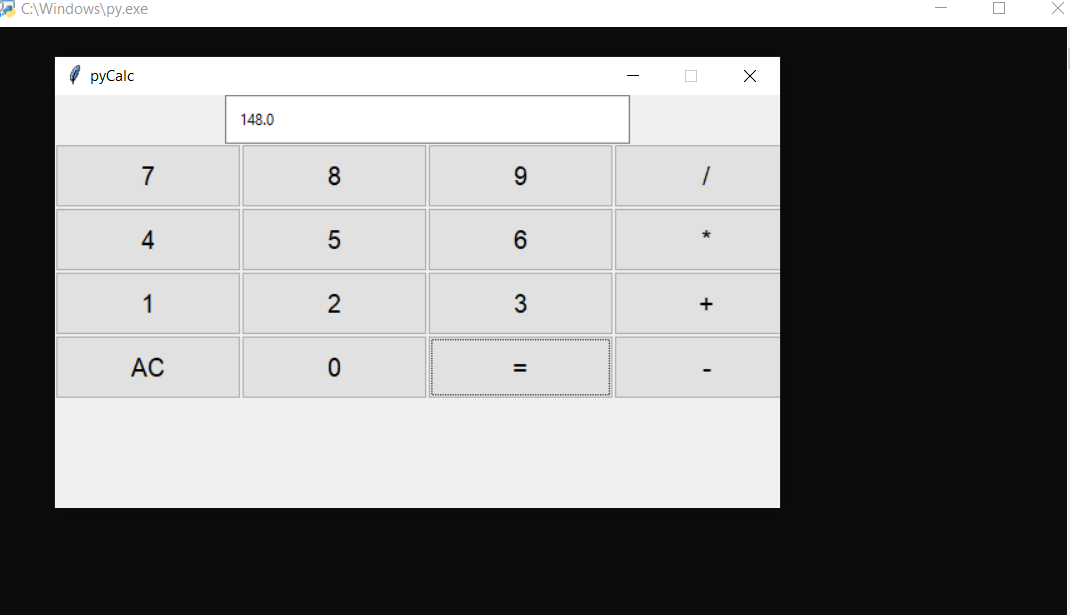## GUI CALCULATOR IN PYTHON WITH SOURCE CODE

The GUI Calculator In Python is a simple project developed using Python. The project contains the numbers, operators, and sign like in normal calculator. So, the user cannot enter the number themselves, they can just click on the numbers they want in the ...

Type : Project

File Size : 5.0 KB# Similar Projects and Reports

#### Calculator With GUI In Python With Source Code

The Calculator With GUI In Python is a simple project developed using Python. The project contains the numbers, operators, and signs like in a normal calculator. So, the user cannot enter the number themselves, they can just click on the numbers they want...

#### GUI CALCULATOR IN PYTHON WITH SOURCE CODE

The GUI Calculator In Python is a simple project developed using Python. The project contains the numbers, operators, and sign like in normal calculator. So, the user cannot enter the number themselves, they can just click on the numbers they want in the ...

#### Python Calculator In GUI With Source Code

The Python Calculator In GUI is a simple project developed using Python. The project contains the numbers, operators, and signs like on a normal calculator. Here, the user can just click on the numbers they want in the calculations. Hence, the user can us...

#### CALCULATOR IN PYTHON WITH SOURCE CODE

This Calculator project In Python is a simple project developed using Python. The project contains the numbers, operators, and sign like in normal calculator. So, the user can enter any number they want in the calculations. Hence, the user can use a simpl...

#### Calculator With Tkinter GUI In Python With Source Code

The Calculator With Tkinter GUI In Python is a simple project developed using Python. The project contains the numbers, operators, and signs like on a normal calculator. Here, the user can just click on the numbers they want in the calculations. Hence, th...

#### BMI Calculator In Python With Source Code

The BMI Calculator In Python is a simple project developed using Python. The project is for the user convenient, for checking your BMI. With the BMI value, you can check whether you have a healthy weight or not. The project file contains a python script ...